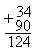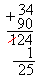Following is an excerpt from
W.Simon,
Mathematical Magic

# A Matter of Age

Over the centuries the number 9 has gained a reputation for having mysterious properties. Its use, in various arithmetical computations, seems to present it with a magic halo. Actually, as the late puzzle genius Sam Lloyd, Senior, pointed out; the number 9 is not imbued with supernatural characteristics ... it is its position as the last single digit in our numerical system which lends itself to many flexible uses. If our system was built on 5 digits, for example, then the number 4 would inherit these characteristics.

I mention this because 9 is an extremely convenient and popularly used number. The following item uses the number 9 as a "key" number, but I will discuss how other numbers may be used if you wish.

An amusing sequence is one in which you determine a person's age although you are given very limited information. There are many systems for doing this, but the method described here is not, to my knowledge, too well known.

Have a spectator (whose age you couldn't possibly know) assist you. He is to perform the following operations while you are turned away from him so that you can't see what he writes:

1. He is to write his age on a slip of paper. (Let us assume that he is 34 years old and thus writes "34" on the paper.)
2. He is to write "your lucky number" beneath his age. Inform him that your lucky number is 90. Thus, he writes 90 beneath his age.
3. He is to add these two numbers together. If this has been done correctly, it will appear as follows:4. He is now instructed to cross out the digit to the left of his total and transplant "whatever this digit is" beneath the remaining numbers in the total. He lastly adds the transplanted number to the number directly above it. If your instructions have been performed correctly, things should appear as follows:5. At this point you mention that your spectator has arrived at a total which has no relation to his age, or to any of the numbers worked with. You request him to tell you what this number is. He will tell you that the total is 25. On the basis of this information you are able to tell the spectator his age! Of course, this should be disclosed in an impressive manner.
6. Your work is quite simple. Upon learning the total (in this case it is 25) you simply add 9 to it. This will give you the spectator's age (25 plus 9 equals 34).

At this point I suggest that you try the above with your own age, or with any two digit number. Follow the indicated steps and add 9 to the total arrived at in step "e." You will return to your age (or the number you started with).

The working behind this is quite novel. At one point you have 90 added in (step "b"), and at another point you have 99 taken away (step "d" actually results in a reduction of 99 from the total). The net result is a subtraction of 9 from your spectator's original starting number ... his age. Thus, when you are told the final total, you need merely add 9 to it to bring it back to its original value.

As mentioned, 9 is a convenient number to use in this stunt. This can be changed simply by changing the number you have the spectator add to his age. For example, if 85 was "your lucky number" and you had spectator add 85 to his age (in step "b") instead of go, then to find spectator's age you would have to add 14 to the total given to you (instead of 9).

As an illustration, using 34 as spectator's age, add 85 to it (34 plus 85 equals 119). The digit to the left, 1, is crossed out and then added to the remaining digits (1 plus 19 equals 2o). Thus, you would be given the total 2o. To this add 14 (secretly) and you return to the original age 34 (20 Plus I4).

Any "lucky number" may be used providing that this number and your spectator's age will total over 100. You simply subtract your "lucky number" from 99 to determine what number you must secretly add to the final total given to you to return the number to its original value. For example, if you choose to have your spectator use 77 as your "lucky number" you would secretly add 22 to the final total (77 subtracted from 99). To illustrate, let us assume that your spectator is 34 and he adds "your lucky number" 77 to his age.

The total would be 111 (77 Plus 34). He would then be instructed to cross out the digit to the left (a 1), and transpose this beneath the remaining digits. The remaining digits (11) would be added to the transposed digit (11 plus 1) and you would be given the total I 2. To this you secretly add 22, which would bring you back to your spectator's age: 34 (12 plus 22).

In showing this to your friends it is important that your spectator clearly understands your instructions. This can be an amusing parlor stunt, and you can vary the "lucky number" as you wish.

The next item, The Fibonacci Series, is quite novel and uses an interesting and little used principle.